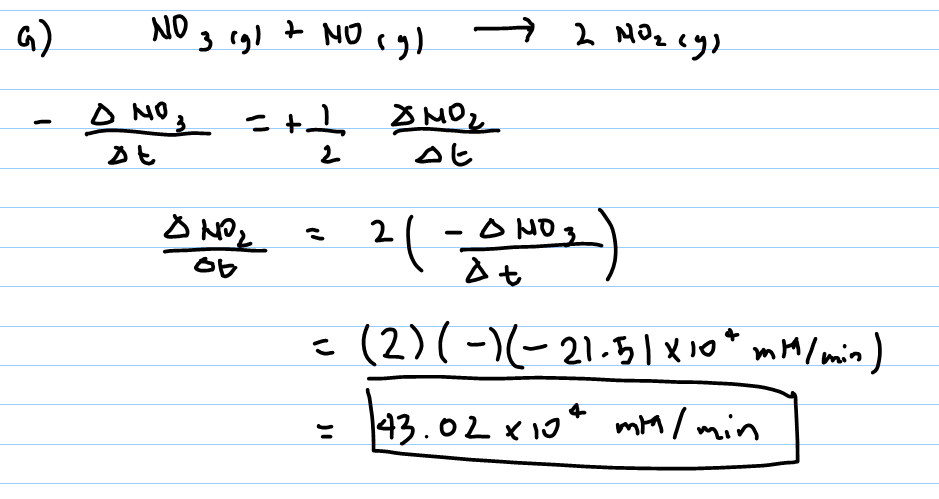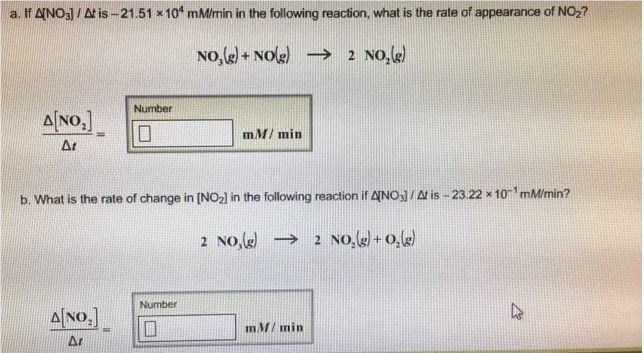# Problem: a. If Δ[NO_3]/Δt is -21.51 x 104 mM/min in the following reaction, what is the rate of appearance of NO2? NO3(g) + NO(g) → 2NO2(g) Δ[NO2]/Δt = b. What is the rate of change in [NO2] in the following reaction if delta [NO3]/Δ t is -23.22 x 10-1 mM/min? 2NO3(g) → 2 NO2(g) + O(g) Δ[NO2]/rΔ =

###### FREE Expert Solution###### Problem Details

a. If Δ[NO_3]/Δt is -21.51 x 104 mM/min in the following reaction, what is the rate of appearance of NO2? NO3(g) + NO(g) → 2NO2(g)

Δ[NO2]/Δt =

b. What is the rate of change in [NO2] in the following reaction if delta [NO3]/Δ t is -23.22 x 10-1 mM/min? 2NO3(g) → 2 NO2(g) + O(g) Δ

[NO2]/rΔ =